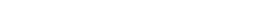Aptitude Questions – Profit and Loss Set 23

Hello Aspirants.

Welcome to Online Quantitative Aptitude section in AffairsCloud.com. Here we are creating question sample in Profit and loss, which is common for all the competitive exams. We have included Some questions that are repeatedly asked in exams!!!

Profit & Loss

1. A milkman buys two cows for Rs. 3000. He sells first cow at a profit of 22% and the second cow at a loss of 8%. What is the SP of second cow if in the whole transaction there is no profit no loss?
1) Rs. 2312
2) Rs. 2024
3) Rs. 2484
4) Rs. 2532
5) None of these
Explanation:2. Sum of CP’s of two cows is Rs. 39, 000. Both the cows are sold at a profit of 20% and 40% respectively with their SP’s being the same. What is the difference of CP’s of both the cows?
1) Rs. 3,000
2) Rs. 2, 000
3) Rs. 1, 500
4) Rs. 2, 500
5) None of these
Explanation:3. A shopkeeper sells his gods at its CP only. But he uses 650 g weight at the place of 1000 g weight for a kg. What is his net profit percentage?
1) 55%
2) 20 1/3%
3) 49 2/3%
4) 53 11/13%
5) None of these
Explanation:4. A seller calculated his intended selling price at 6% profit on the cost of a product. However, owing to some mistake while selling, the units and tens digits of the selling price got interchanged. This reduced the profit by Rs. 180 and profit percentage to 2.4%. What is the cost price of the product?
1) Rs. 4500
2) Rs. 5000
3) Rs. 4750
4) Rs. 6000
5) None of these
Explanation:5. Jim sells a book to Carrey at a profit of 20% and Carrey sells this book to Sid at a profit of 25%. Now Sid sells this book at a loss of 10% to Simba. At what percentage loss should Simba sells this book now so that his SP becomes equal to Jim’s CP?
1) 26.68%
2) 25.92%
3) 58.66
4) Cannot be determined
5) None of these
Explanation:6. A fruit vendor professes to sell fruits at the cost price, but uses false weights. He gains 40% in this manner. What weight does he substitute for one kilogram?
1) 745 1/3 g
2) 750 g
3) 714 2/7 g
4) 800 g
5) None of these
Answer – 3) 714 2/7 g
Explanation:7. A shopkeeper marks up his goods by 20% and then gives a discount of 20%. Besides he cheats both his supplier and customer by 100 g, i.e., he takes 1100 g from his supplier and sells only 900 g to his customer. What is his net proﬁt percentage? (Rounded off to two decimal points)
1) 24.5%
2) 17.33%
3) 25%
4) 32.5%
5) None of these
Explanation:8. Some mangoes are purchased at the rate of 8 mangoes/Rs and some more mangoes at the rate of 6 mangoes/Rs, investment being equal in both the cases. Now, the whole quantity is sold at the rate of 3.5 mangoes/Rs What is the net percentage proﬁt/loss?
1) 100% proﬁt
2) 60% loss
3) 80% loss
4) no proﬁt/no loss
5) None of these
Explanation:9. A man would gain 25% by selling a chair for Rs. 47.5 and would gain 15% by selling a table for Rs. 57.5. He sells the chair for Rs. 45; what is the least price for which he must sell the table to avoid any loss on the two together?
1) Rs. 41.2
2) Rs. 48.5
3) Rs. 42.5
4) Rs. 43
5) Cannot be determined
Explanation:10. A bookseller marks his books at an advance of 69% on the actual cost of producton He allows a discount of 15% and also given a copy free for every dozen sold aa time. What rate per cent proﬁt does the book seller make, if books are sold in lots of 24? (find the approximate value)
1) 38%
2) 47%
3) 24%
4) None of these
5) Cannot be determined